Scroll

# Ranking Within Columns & Edges

Follow
 Summary This article provides examples of how to rank on any calculation across your columns and edges. It also provides an example work around if you need to work with edge suppression Applies to Askia Analyse. Written for Data Processors, Programmers and Analysts. Keywords Ranking, Edge suppression, Column suppression, Edge index, Column index, Calculation, Table, Arithmetic, Script, Cleaning scripts, Colour coded, FindIndexIn, Sort.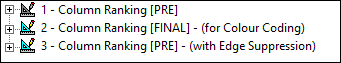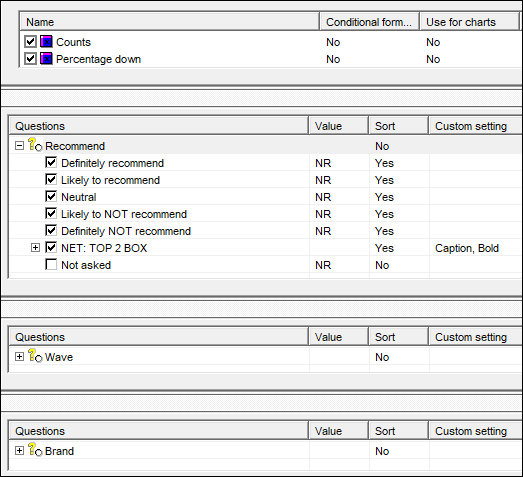We want to achieve two types of ranking:

1. Rank the brands across waves based on Top 2 Box % (Rank within edges)
2. Rank the waves within a brand based on Top 2 Box % (Rank within columns)

Take a look at the results of the table definition, Column Ranking [PRE]: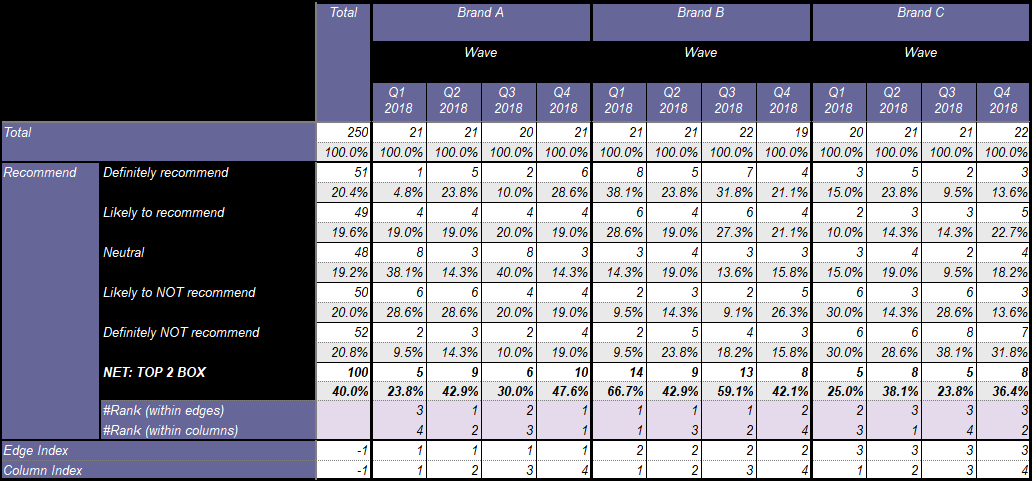The syntax for achieving these is outlined below. The method is first to create a ranking array in each cell and then extract the correct rank for the wave or brand using the edge or column index.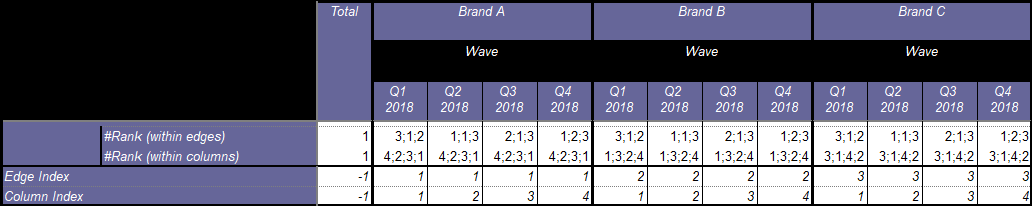Firstly the syntax for ranking brands across waves: #Rank (within edges).

```Dim numSetT2B = 0
Dim j = 0
Dim k = 0
For j = CurrentTable.StartY To CurrentTable.MaxY
For k = 1 to CurrentTable.StartX                                              ' scan all columns until start of data for "Top 2 BOX"
If InStr( "TOP 2 BOX", CurrentTable.GetCell(k,j).Text) > 0 Then
numSetT2B = j+1                                                           ' find y-coord of "Top 2 BOX" label, +1 to find the % row
Endif
Next k
Next j

Dim nColId = CurrentCell.Column.Index

Dim arrSet = {}
Dim arrRank = {}
Dim i = 0
For i = CurrentTable.StartX To CurrentTable.MaxX
If  nColId = CurrentTable.GetCell(i,numSetT2B).Column.Index Then             ' For all matching column indexes
arrSet.Insert(CurrentTable.GetCell(i,numSetT2B).Value)                       ' add the values of the Top 2 BOX row to an array (of size 3 because we have 3 edge responses)
Endif
Next i

Dim h = 0
For h = CurrentTable.StartX To CurrentTable.MaxX
If  nColId = CurrentTable.GetCell(h,numSetT2B).Column.Index Then
arrRank.Insert(FindIndexIn(arrSet.SortDesc(),CurrentTable.GetCell(h,numSetT2B).Value))      ' Sort descending each array of values for each matching column index and find the rank of the current value in that array
EndIf
Next h

Return arrRank[CurrentCell.Edges.Index]                                         ' use the edge index to extract the correct rank for the brand across waves from the array
```

The syntax for ranking waves within a brand: #Rank (within columns), is much the same. You just need to swap any references to columns with references to edges e.g.

Line 12: - Dim nColId = CurrentCell.Column.Index becomes: Dim nEdgeId = CurrentCell.Edges.Index

Line 30: - Return arrRank[CurrentCell.Edges.Index] becomes: Return arrRank[CurrentCell.Column .Index]

The only other swaps are on lines 18 & 25.

Appendix I:

If you're interested, colour-coding of the ranks and headers are possible by script to better help identify by eye which rank belongs to which brand or wave: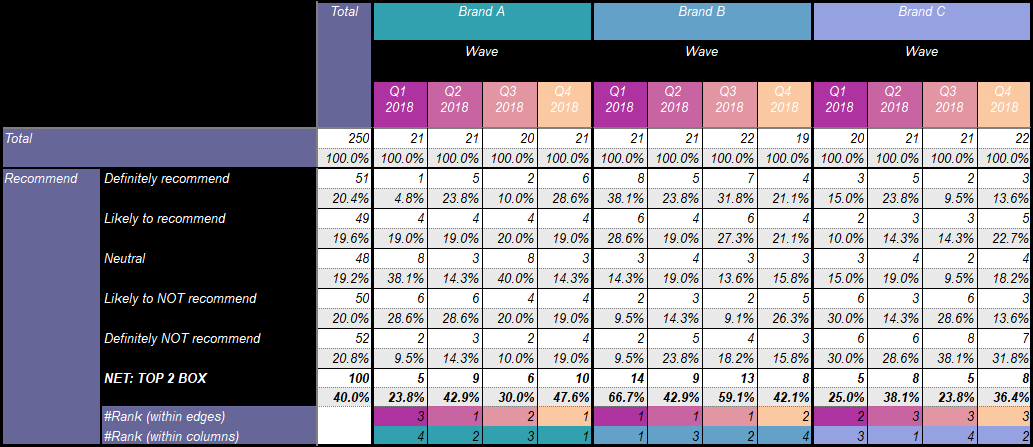This can be seen in the tab definition: Column Ranking [FINAL]. The script requires knowledge of the column and edge indexes. However, since CurrentCell is not a valid keyword in cleaning scripts and these indexes are not showing in the current table, we need to get them from another table where they are showing, Column Ranking [PRE], highlighted in the script here.

Appendix II:

Let's assume we have have a wave for which there is no data and edge suppression: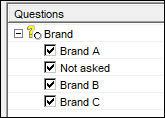This will cause the current set-up to malfunction because CurrentCell.Edges.Index gives the index whether it's suppressed or not and the current set-up supposes all edge responses appear in the table. You can immediately see a problem in the rankings (boxed in red in the screenshot below).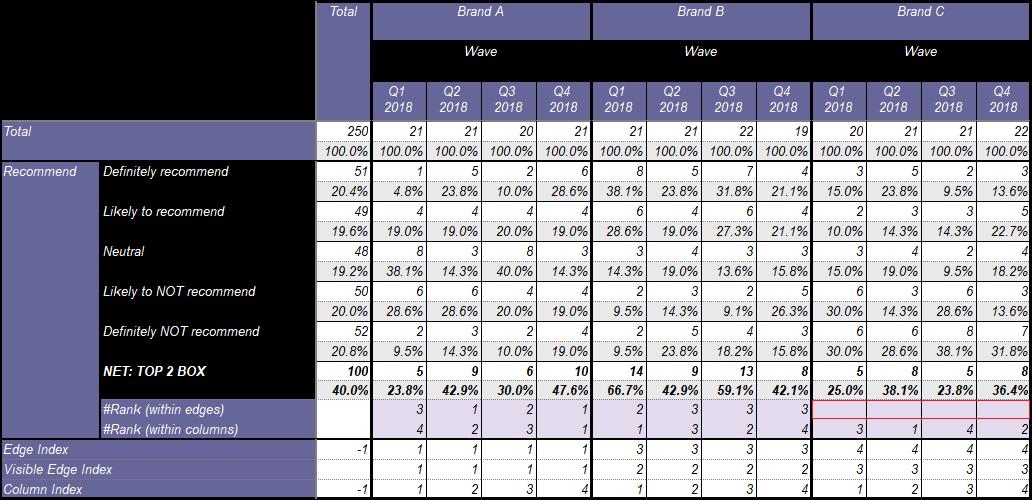The way to work around this is substitute

CurrentCell.Edges.Index

with another piece of syntax which counts/inexes only the visible edges. You can see I've set this up in the Visible Edge Index calculation:

`Dim numVisColResps = CurrentTable.GetCell(CurrentTable.MaxX,CurrentTable.MaxY).Column.Index         ' find the last column of the table and check its col indexDim numVisibleEdgeIndex = (CurrentCell.X - CurrentTable.StartX) / numVisColRespsReturn numVisibleEdgeIndex.Ceil()`

Add the first two lines to your ranking script and substitute the visible edge index value in to the return value: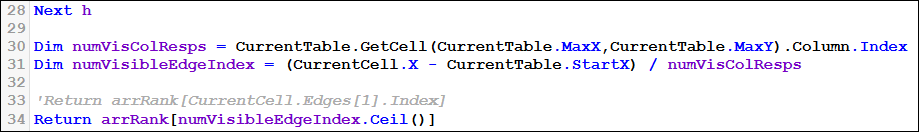Then it works: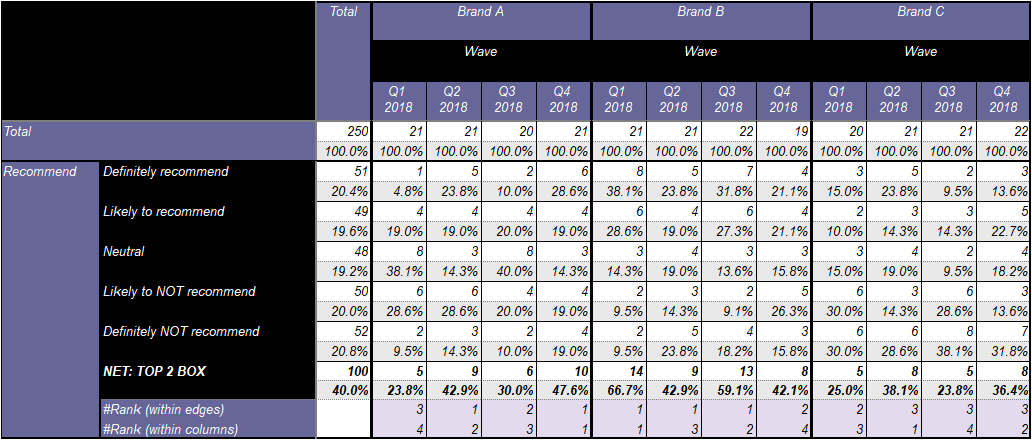Note this does not work with both edge and column suppression. This method supposes the column question always has the same number of unsuppressed responses within each edge (4 in this case). If you require column suppression bu no edge suppression then either swap them around in your table definition or contact askia support.